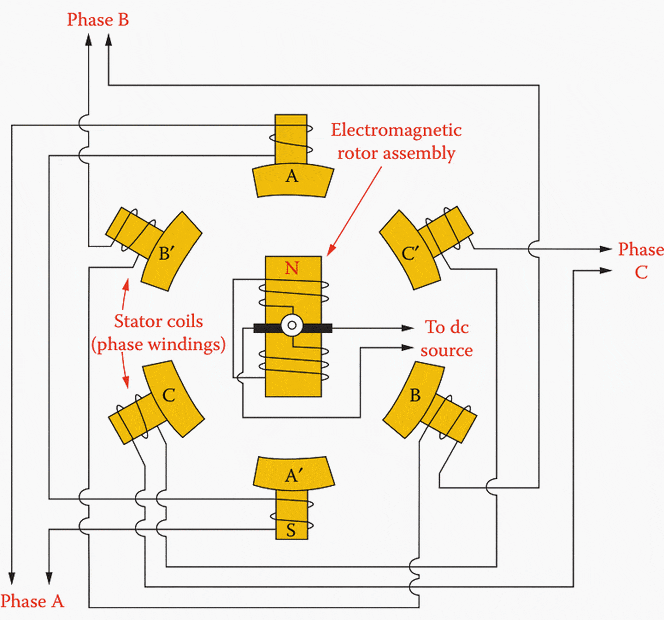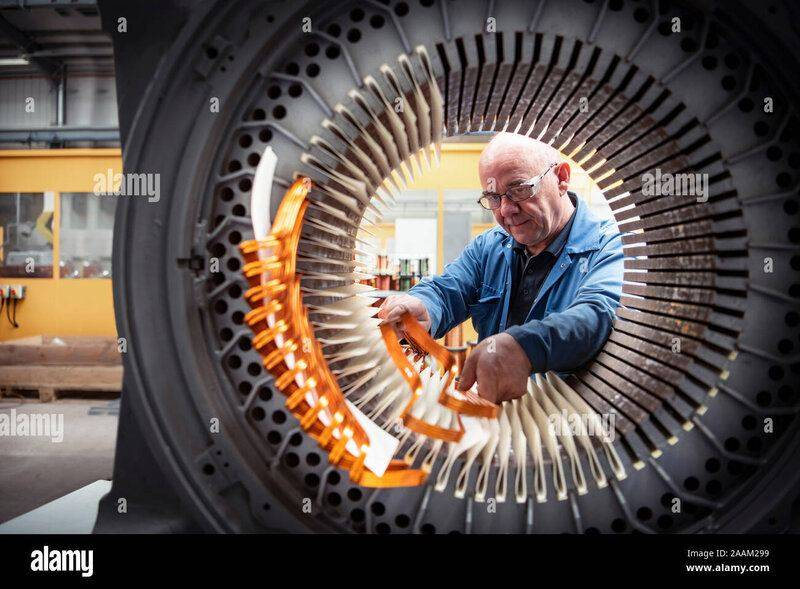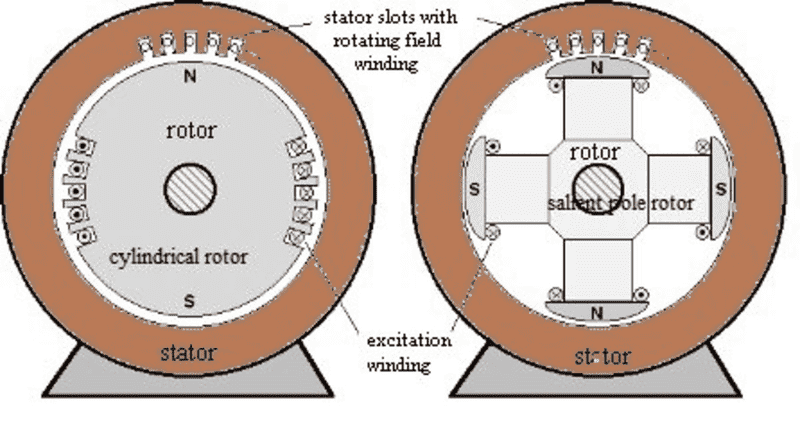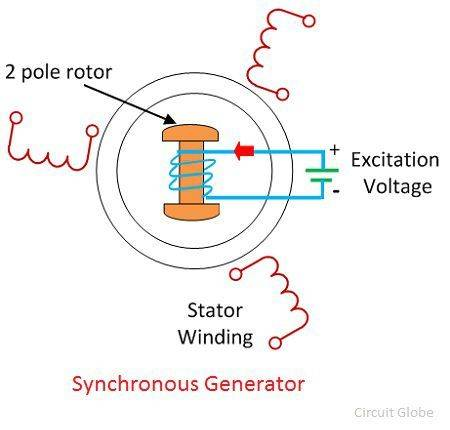# How are coils arranged in a power station generator?

• Jimmy87

#### Jimmy87

Hi,

I wanted to know a couple of things regarding the construction of the stator and rotor of a generator in a power station. Firstly, how the copper windings inside the stator are arranged. I understand it’s a three phase winding where each phase has a set of two coils like this:Source: https://electrical-engineering-portal.com/power-generation-systems

Why are there two sets of coils per phase instead of one set of coils for each phase? Also, this is just a diagram and wondered how it translates to an actual diagram like this:Source: https://www.alamy.com/electrical-en...rical-engineering-factory-image333584421.html

How do all these many windings he is putting in equate to the first diagram of three pairs of coils. They look so dissimilar.

The other query I had is what do they use to power the rotor part of the generator which is essentially an electromagnet so will require an electrical input?

Thanks

Both very good questions.

Most power plants use synchronous generators like the ones you showed in the diagram. Synchronous generators can be of the salient pole type, or the round rotor type. Your diagram shows salient poles.

For 60 Hz power, if you have one pair of poles, the rotor must spin at 3600 RPM. If you have 2 pole pairs, the speed is only 1800 RPM. Nuclear turbines have longer blades because of lower temperatures. They run at 1800 RPM. Some hydro plants run at as little as 180 RPM. That means 20 pole pairs.

Round rotors make the windings go the other way. Instead of windings around the pole, they go around the entire rotor.We use DC field windings rather than permanent magnets in the rotor so that we can vary the voltage. Increasing the voltage while holding power constant causes more VARS (Volt Amperes Reactive) to be injected into the grid. That tends to increase voltage everywhere in the power grid. With a permanent magnet synchronous generator, you have no way to adjust the voltage. See the PF Insights Article for elaboration on that point.
https://www.physicsforums.com/insights/ac-power-analysis-part-2-network-analysis/

To understand power and the power grid, you really must understand the difference between voltage angle and voltage magnitude, plus real and reactive power. Power engineers learn, "Angle determines power flow, voltage magnitude determines VAR flow." Its a very simple concept, but you must learn a bit of math first. I have another Insights article for that.
https://www.physicsforums.com/insights/ac-power-analysis-part-1-basics/

Last edited:
••Baluncore, russ_watters, dlgoff and 2 others
Why are there two sets of coils per phase instead of one set of coils for each phase?
I only see one set of coils per phase. In my opinion a "set" of coils is a pair. So a total of 6, which is what the diagram shows.

•Jimmy87
I only see one set of coils per phase. In my opinion a "set" of coils is a pair. So a total of 6, which is what the diagram shows.
Yes but could have three single sets of coils like the one below? Is there a reason they use them in a pair like this giving 6 total?Both very good questions.

Most power plants use synchronous generators like the ones you showed in the diagram. Synchronous generators can be of the salient pole type, or the round rotor type. Your diagram shows salient poles.

For 60 Hz power, if you have one pair of poles, the rotor must spin at 3600 RPM. If you have 2 pole pairs, the speed is only 1800 RPM. Nuclear turbines have longer blades because of lower temperatures. They run at 1800 RPM. Some hydro plants run at as little as 180 RPM. That means 20 pole pairs.

Round rotors make the windings go the other way. Instead of windings around the pole, they go around the entire rotor.
View attachment 303384

We use DC field windings rather than permanent magnets in the rotor so that we can vary the voltage. Increasing the voltage while holding power constant causes more VARS (Volt Amperes Reactive) to be injected into the grid. That tends to increase voltage everywhere in the power grid. With a permanent magnet synchronous generator, you have no way to adjust the voltage. See the PF Insights Article for elaboration on that point.
https://www.physicsforums.com/insights/ac-power-analysis-part-2-network-analysis/

To understand power and the power grid, you really must understand the difference between voltage angle and voltage magnitude, plus real and reactive power. Power engineers learn, "Angle determines power flow, voltage determines VAR flow." Its a very simple concept, but you must learn a bit of math first. I have another Insights article for that.
https://www.physicsforums.com/insights/ac-power-analysis-part-1-basics/
Wow thanks that’s really interesting. I assumed in the UK all the generators rotated at 50Hz I didn’t realize they rotated at different rates depending on the number of rotor poles.

How are the stator windings arranged? In my OP it doesn’t at all look like that guy is wiring 3 sets of coils for each phase; looks like hundreds of different coils.

I have just found this clip to better explain what I mean:

So at 1min 40s they start adding a set of stator coils for each phase which makes sense as they add three. At 3mins 50s they suddenly have hundreds of coils and I don’t see how that still amounts to only three phases with so many coils?

I have just found this clip to better explain what I mean
Thanks. I am really bad describing things like that with words.

Wow thanks that’s really interesting. I assumed in the UK all the generators rotated at 50Hz I didn’t realize they rotated at different rates depending on the number of rotor poles.
Just think, 50 pole pairs per second for all the generators regardless of RPM.

Wow thanks that’s really interesting. I assumed in the UK all the generators rotated at 50Hz I didn’t realize they rotated at different rates depending on the number of rotor poles.

How are the stator windings arranged? In my OP it doesn’t at all look like that guy is wiring 3 sets of coils for each phase; looks like hundreds of different coils.
You are confused because you just look at the man assembling the stator so it seems like "hundreds of coils" but in the end remember those many coils get electrically connected together in pairs. After this is done they form the final coil arrangement and then what was originally many coils are now just a single coil connected together. Repeat this for the other coils and you end up with 3 or 4 wires coming out of the generator depending on the type of wiring whether delta or star.

By the way the electrical 120 degree angle separation of the sine waves of each phase in a 3 phase system directly corresponds to the physical layout of the coils and rotor pole pairs within a 3 phase generator.
If you were to take a physical 3 phase generator and rewind it in some crazy way so that the stator coils would have uneven distance between them that would also show in the electrical output sine wave.